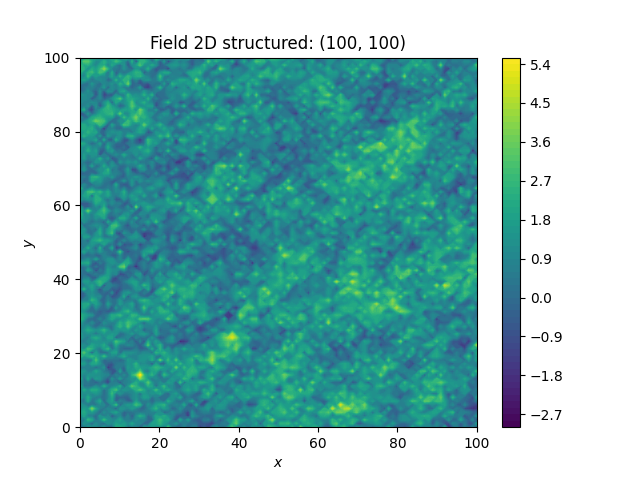# TPL Stable¶

GSTools also implements truncated power law variograms, which can be represented as a superposition of scale dependant modes in form of standard variograms, which are truncated by a lower-and an upper length-scale.

This example shows the truncated power law (TPLStable) based on the Stable covariance model and is given bywith Stable modes on each scale:which gives Gaussian modes for alpha=2 or Exponential modes for alpha=1.

Forthis results in:import numpy as np
import gstools as gs

x = y = np.linspace(0, 100, 100)
model = gs.TPLStable(
dim=2,  # spatial dimension
var=1,  # variance (C is calculated internally, so variance is actually 1)
len_low=0,  # lower truncation of the power law
len_scale=10,  # length scale (a.k.a. range), len_up = len_low + len_scale
nugget=0.1,  # nugget
anis=0.5,  # anisotropy between main direction and transversal ones
angles=np.pi / 4,  # rotation angles
alpha=1.5,  # shape parameter from the stable model
hurst=0.7,  # hurst coefficient from the power law
)
srf = gs.SRF(model, mean=1.0, seed=19970221)
srf.structured([x, y])
srf.plot()


Total running time of the script: ( 0 minutes 15.473 seconds)

Gallery generated by Sphinx-Gallery